Home > Engineering> Mechanical Engineering

6 . The time of flight (t) of a projectile on an upward inclined plane is(where u = Velocity of projection, α = Angle of projection, and β = Inclination of the plane with the horizontal.)
 A.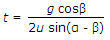B.C.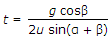D.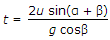7 . The uniIf the masses of both the bodies, as shown in the below figure, are reduced to 50 percent, then tension in the string will be t of angular acceleration is
 A.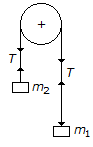B. same C. half D. double

8 . If a number of forces are acting at a point, theirresultant will be inclined at an angle θ with the horizontal, such that
 A. tan θ = ∑H/∑V B. tan θ = ∑V/∑H C. tan θ = ∑Vx∑H D.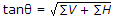9 . The range of a projectile is maximum, when the angle of projection is
 A. 30° B. 45° C. 60° D. 90°

10 . The mechanical advantage of a lifting machine is the ratio of
 A. distance moved by effort to the distance moved by load B. load lifted to the effort applied C. output to the input D. all of the above

Loading...

### Aptitude

© 2016-2021 by GK INDIA ONLINE